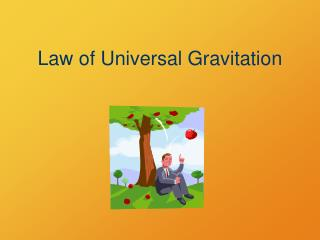DownloadDownload PresentationLaw of Universal Gravitation

# Law of Universal Gravitation

Download Presentation## Law of Universal Gravitation

- - - - - - - - - - - - - - - - - - - - - - - - - - - E N D - - - - - - - - - - - - - - - - - - - - - - - - - - -
##### Presentation Transcript

1. Law of Universal Gravitation

2. Newton’s Universal Law of Gravity • Legend has it that Newton was struck on the head by a falling apple while napping under a tree. This prompted Newton to imagine that all bodies in the universe are attracted to each other in the same way that the apple was attracted to the Earth. • Newton analyzed astronomical data on the motion of the Moon around the Earth and stated that the law of force governing the motion of the planets has the same mathematical form as the force law that attracts the falling apple to the Earth.

3. Newton’s Universal Law of Gravity • Newton’s law of gravitation: every particle in the universe attracts every other particle with a force that is directly proportional to the product of their masses and inversely proportional to the square of the distance between them. • If the particles have masses m1 and m2 and are separated by a distance d, the magnitude of the gravitational force is:

4. Newton’s Universal Law of Gravity • G is the universal gravitational constant, which has been measured experimentally as 6.67 x 10-11 . • The distance d between m1 and m2 is measured from the center of m1 to the center of m2. d

5. By Newton’s third law, the magnitude of the force exerted by m1 on m2 is equal to the force exerted by m2 on m1, but opposite in direction. These gravitational forces form an action-reaction pair. Newton’s Universal Law of Gravity d

6. Newton’s Law of Universal Gravitation d Source: Griffith, The Physics of Everyday Phenomena, 4th ed. Equal, opposite forces pull the objects together

7. m1m2 F = G d2 Newton’s Law of Universal Gravitation Force between two objects: m1, m2: masses of the objects d: distance between objects’ centers of mass G: universal gravitational constant, 6.67  10–11 Nm2/kg2

8. m1m2 F = G d2 Properties of Gravity • direction is toward the other object • magnitude decreases as (distance)2 increases • never becomes zero

9. Practice Problem 1 • Tom has a mass of 70.0-kg and Sally has a mass of 50.0-kg. Tom and Sally are standing 20.0m apart on the dance floor. Sally looks up and sees Tom. She feels an attraction. If the attraction is gravitational, find its size. Assume that both Tom and Sally can be replaced by spherical masses. • 5.838 x 10-10 N

10. Practice Problem 2 • What is the gravitational attraction between two spheres (msphere = 1.67 X 10-27 kg) at a distance of 5.0 X 10-15 m? • 7.44 x10-36 N

11. Practice Problem 3 • A 1.0-kg mass weighs 9.8 N on earth’s surface, and the radius of Earth is roughly 6.4 x 106 meters. Calculate the mass of Earth. • 9.8 N = (6.6710-11 Nm2/kg2)(1.0kg)(m2) (6.4x106 m)2 • 6.02 x 1024 kg

12. Book problems • pg. 194 #9, 11, 33, 35, 36, 37, 53(only part a)